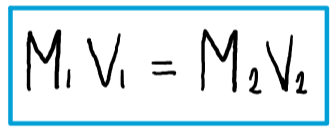# Problem: If 0.1718 L of a 0.3556-M C3H7OH solution is diluted to a concentration of 0.1222 M, what is the volume of the resulting solution?

###### FREE Expert Solution

The equation for dilution is given by:where M1 and V1 are the concentration and volume of the initial solution, respectively;

and M2 and V2 are the concentration and volume of the diluted solution, respectively.

97% (28 ratings)###### Problem Details

If 0.1718 L of a 0.3556-M C3H7OH solution is diluted to a concentration of 0.1222 M, what is the volume of the resulting solution?